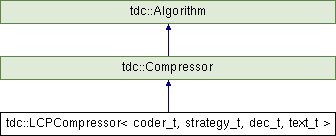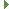tudocomp – The TU Dortmund Compression Framework
tdc::LCPCompressor< coder_t, strategy_t, dec_t, text_t > Class Template Reference

Factorizes the input by finding redundant phrases in a re-ordered version of the LCP table. More...

`#include <LCPCompressor.hpp>`

Inheritance diagram for tdc::LCPCompressor< coder_t, strategy_t, dec_t, text_t >:## Public Member Functions

LCPCompressor (Env &&env)
Construct the class with an environment. More...

virtual void compress (Input &input, Output &output) override
Compress the given input to the given output. More...

virtual void decompress (Input &input, Output &output) override
Decompress the given input to the given output. More...Public Member Functions inherited from tdc::Compressor
virtual ~Compressor ()=default

Compressor (Compressor const &)=default

Compressor (Compressor &&)=default

Compressoroperator= (Compressor const &)=default

Compressoroperator= (Compressor &&)=default

Compressor (Env &&env)
Construct the compressor with an environment. More...Public Member Functions inherited from tdc::Algorithm
virtual ~Algorithm ()=default

Algorithm (Algorithm const &)=default

Algorithm (Algorithm &&)=default

Algorithmoperator= (Algorithm const &)=default

Algorithmoperator= (Algorithm &&)=default

Algorithm (Env &&env)
Instantiates an algorithm in the specified environment. More...

Envenv ()

const Envenv () const

## Static Public Member Functions

static Meta meta ()

## Detailed Description

### template<typename coder_t, typename strategy_t, typename dec_t, typename text_t = TextDS<>> class tdc::LCPCompressor< coder_t, strategy_t, dec_t, text_t >

Factorizes the input by finding redundant phrases in a re-ordered version of the LCP table.

Definition at line 83 of file LCPCompressor.hpp.

## ◆ LCPCompressor()

template<typename coder_t , typename strategy_t , typename dec_t , typename text_t = TextDS<>>
 tdc::LCPCompressor< coder_t, strategy_t, dec_t, text_t >::LCPCompressor ( Env && env )
inline

Construct the class with an environment.

Definition at line 98 of file LCPCompressor.hpp.

## ◆ compress()

template<typename coder_t , typename strategy_t , typename dec_t , typename text_t = TextDS<>>
 virtual void tdc::LCPCompressor< coder_t, strategy_t, dec_t, text_t >::compress ( Input & input, Output & output )
inlineoverridevirtual

Compress the given input to the given output.

Parameters
 input The input. output The output.

Implements tdc::Compressor.

Definition at line 100 of file LCPCompressor.hpp.

## ◆ decompress()

template<typename coder_t , typename strategy_t , typename dec_t , typename text_t = TextDS<>>
 virtual void tdc::LCPCompressor< coder_t, strategy_t, dec_t, text_t >::decompress ( Input & input, Output & output )
inlineoverridevirtual

Decompress the given input to the given output.

Parameters
 input The input. output The output.

Implements tdc::Compressor.

Definition at line 140 of file LCPCompressor.hpp.

## ◆ meta()

template<typename coder_t , typename strategy_t , typename dec_t , typename text_t = TextDS<>>
 static Meta tdc::LCPCompressor< coder_t, strategy_t, dec_t, text_t >::meta ( )
inlinestatic

Definition at line 85 of file LCPCompressor.hpp.

The documentation for this class was generated from the following file: